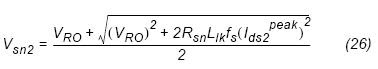# Electronic Device And Electronic Circuit

Data of electronic device , PCB Design and electronic circuitWeb basicelectronic.blogspot.com

## Wednesday, March 25, 2009

### Design the MOSFET RCD Snubber Circuit

When the power MOSFET is turned off, there is a high
voltage spike on the drain due to the transformer leakage
inductance. This excessive voltage on the MOSFET may
lead to an avalanche breakdown and eventually failure of the
FPS. Therefore, it is necessary to use an additional network
to clamp the voltage.

The RCD snubber circuit and MOSFET drain voltage
waveform are shown in Figure 10 and 11, respectively. The
RCD snubber network absorbs the current in the leakage
inductance by turning on the snubber diode (Dsn) once the
MOSFET drain voltage exceeds the voltage of node X as
depicted in Figure 10. In the analysis of snubber network, it
is assumed that the snubber capacitor is large enough that its
voltage does not change significantly during one switching
cycle. The snubber capacitor used should be ceramic or a
material that offers low ESR. Electrolytic or tantalum
capacitors are unacceptable due to these reasonCircuit diagram of the snubber network

The first step in designing the snubber circuit is to determine
the snubber capacitor voltage at the minimum input voltage
and full load condition (Vsn). Once Vsn is determined, the
power dissipated in the snubber network at the minimum
input voltage and full load condition is obtained aswhere Ids-peak is specified in equation (8), fs is the FPS
switching frequency, Llk is the leakage inductance, Vsn is the
snubber capacitor voltage at the minimum input voltage and
full load condition, VRO is the reflected output voltage and
Rsn is the snubber resistor. Vsn should be larger than VRO
and it is typical to set Vsn to be 2~2.5 times VRO. Too small a
Vsn results in a severe loss in the snubber network as shown
in equation (23). The leakage inductance is measured at the
switching frequency on the primary winding with all other
windings shorted.
Then, the snubber resistor with proper rated wattage should
be chosen based on the power loss. The maximum ripple of
the snubber capacitor voltage is obtained aswhere fs is the FPS switching frequency. In general, 5~10%
ripple of the selected capacitor voltage is reasonable.
The snubber capacitor voltage (Vsn) of equation (26) is for
the minimum input voltage and full load condition. When
the converter is designed to operate in CCM under this
condition, the peak drain current together with the snubber
capacitor voltage decrease as the input voltage increases as
shown in Figure 11. The peak drain current at the maximum
input voltage and full load condition (Ids2 peak) is obtained aswhere Pin, and Lm are specified in equations (1) and (6),
respectively and fs is the FPS switching frequency.
The snubber capacitor voltage under maximum input voltage
and full load condition is obtained aswhere fs is the FPS switching frequency, Llk is the primary
side leakage inductance, VRO is the reflected output voltage
and Rsn is the snubber resistor.

From equation (26), the maximum voltage stress on the
internal MOSFET is given bywhere VDC max is specified in equation (3). Check if Vds
max is below 85% of the rated voltage of the
MOSFET (BVdss) as shown in Figure 12. The voltage rating
of the snubber diode should be higher than BVdss. Usually,
an ultra fast diode with 1A current rating is used for the
snubber network.

In the snubber design in this section, neither the lossy
discharge of the inductor nor stray capacitance is considered.
In the actual converter, the loss in the snubber network is

Less than the designed value due to this effectsSource
Design Considerations for Battery Charger Using
Green Mode Fairchild Power Switch (FPSTM)

http://www.fairchildsemi.com/an/AN/AN-4138.pdf

Labels: